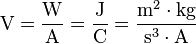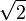# Volt facts for kids

Kids Encyclopedia Facts
For the electric plug-in hybrid concept car, see Chevrolet Volt.
For the record label, see Volt Records

The volt (symbol: V) is the SI derived unit of electric potential difference or electromotive force. It is named in honor of the Italian physicist Alessandro Volta (1745–1827), who invented the voltaic pile, the first chemical battery.

## Definition

The volt is defined as the potential difference across a conductor when a current of one ampere dissipates one watt of power. Hence, it is the base SI representation m2 · kg · s−3 · A−1, which can be equally represented as one joule of energy per coulomb of charge, J/C.$\mbox{V} = \dfrac{\mbox{W}}{\mbox{A}} = \dfrac{\mbox{J}}{\mbox{C}} = \dfrac{\mbox{m}^2 \cdot \mbox{kg}}{\mbox{s}^{3} \cdot \mbox{A}}$

## Hydraulic analogy

In the hydraulic analogy sometimes used to explain electric circuits by comparing them to water-filled pipes, voltage is likened to water pressure - it determines how fast the electrons will travel through the circuit. Current (in amperes), in the same analogy, is a measure of the volume of water that flows past a given point, the rate of which is determined by the voltage, and the total output measured in watts. The equation that brings all three components together is: volts × amperes = watts

## Common voltages

Nominal voltages of familiar sources:

• Nerve cell action potential: around 30 mV
• Single-cell, rechargeable NiMH or NiCd battery: 1.2 V
• Mercury battery 1.355 V
• Single-cell, non-rechargeable alkaline battery (e.g. AAA, AA, C and D cells): 1.5 V
• Lithium polymer rechargeable battery: 3.75 V
• Transistor-transistor logic (TTL) power supply: 5 V
• PP3 battery: 9 V
• Automobile electrical system: 12 V (nominal)
• Household mains electricity: 230 V RMS in Europe, Australia, Asia and Africa, 120 V RMS in North America, 100 V RMS in Japan (see List of countries with mains power plugs, voltages and frequencies)
• Rapid transit third rail: 600 to 700 V (see List of current systems for electric rail traction)
• High speed train overhead power lines: 25 kV RMS at 50 Hz, but see List of current systems for electric rail traction for exceptions.
• High voltage electric power transmission lines: 110 kV RMS and up (1150 kV RMS is the record as of 2005)
• Lightning: Varies greatly, often around 100 MV.

Note: Where 'RMS' (root mean square) is stated above, the peak voltage is$\sqrt{2}$ times greater than the RMS voltage for a sinusoidal signal.

## History of the volt

In 1800, as the result of a professional disagreement over the galvanic response advocated by Luigi Galvani, Alessandro Volta developed the so-called Voltaic pile, a forerunner of the battery, which produced a steady electric current. Volta had determined that the most effective pair of dissimilar metals to produce electricity was zinc and silver. In the 1880s, the International Electrical Congress, now the International Electrotechnical Commission (IEC), approved the volt for electromotive force. The volt was defined as the potential difference across a conductor when a current of one ampere dissipates one watt of power.

## Images for kidsVolt Facts for Kids. Kiddle Encyclopedia.